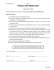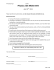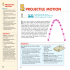# P. LeClair - The University of Alabama

## Transcription

P. LeClair - The University of Alabama
```University of Alabama
Department of Physics and Astronomy
PH 105 LeClair
Summer 2015
Week 2 Homework
Instructions:
1. There are problems assigned for each day of class.
2. The following class, you will turn in the one problem the instructor requests.
3. Only the chosen problem for the day is graded.
5. You may collaborate, but everyone must turn in their own work.
Problems for 2 June (due 3 June)
1. A projectile is launched with initial velocity ~vi from the start of a ramp, with the ramp making
an angle ϕ with respect to the horizontal. The projectile is launched with an angle θ > ϕ with
respect to the horizontal. (a) At what position along the ramp does the projectile land? (b) What
angle θ maximizes the distance the particle makes it along the ramp (your answer will be in terms
of the angle ϕ? Note no numeric solution is required.
⃗vi
θ ϕ
Figure 1: A projectile is launched onto a ramp.
2. In the figure below, a slab of mass m1 = 40 kg rests on a frictionless floor, and a block of mass
m2 = 10 kg rests on top of the slab. Between block and slab, the coefficient of static friction is 0.60,
and the coefficient of kinetic friction is 0.40. A horizontal force ~
F of magnitude 100 N begins to pull
directly on the block, as shown. What are the resulting accelerations of (a) the block, and (b) the
slab?
Figure 2: Problem 2
3. Three boxes are pushed with force F across a frictionless table as shown in the figure below. Let
N1 be the normal force between the left two boxes, and N2 the normal force between the right two
boxes. What are the relative sizes of F, N1 , and N2 ? (I.e., set up an inequality relating the three,
F
m
2m
3m
Figure 3: Problem 3
Problems for 3 June (due 4 June)
4. You have two masses m1 and m2 hanging from a pulley, which you support by holding a
string connected to the pulley’s axis. If you have your eyes closed and think that you are instead
supporting a single mass M at rest, what is M in terms of m1 and m2 ? Is it simply equal to
m 1 + m2 ?
(hand)
m1
m2
5. In the figure below, blocks A and B have weights of 44 N and 22 N, respectively. (a) Determine
the minimum weight of block C to keep A from sliding if µs between the table and A is 0.20. (b)
Block C is suddenly lifted off A. What is the acceleration of block A if µk between A and the table
is 0.15?
Problems for 4 June (due 5 June)
6. A circular rope with radius R and mass density λ (kg/m) lies on a frictionless table and rotates
around its center, with all points moving at speed v. What is the tension in the rope? Hint:
consider the net force on a small piece of rope that subtends an angle θ.
7. Consider the machine shown below. The masses are held at rest and then released. In terms of
m1 and m2 , what should M be so that m1 doesn’t move? What relationship must hold between
m1 and m2 so that such an M exists?
m1
m2
M
Problems for 5 June (due 8 June)
8. In the figure below, a small block of mass m = 0.032 kg can slide along the frictionless loop-theloop, with loop radius R = 12 cm. The block is released from rest at a point P, at height h = 5.0R
above the bottom of the loop. How much work does the gravitational force do on the block as
the block travels from point P to (a) point Q and (b) the top of the loop? If the gravitational
potential energy of the block-Earth system is taken to be zero at the bottom of the loop, what is
that potential energy when the block is (c) at point P, (d) at point Q, and (e) at the top of the
loop? (f ) If, instead of merely being released, the block is given some initial speed downward along
the track, do the answers to (a) through (e) increase, decrease, or remain the same?
Figure 4: A block slides along a loop-the-loop.
9. A block with mass m starts from rest and slides down a plane inclined at angle θ. The coefficient
of kinetic friction is µ. What is the block’s speed after it has traveled a distance d down the plane,
assuming that it does indeed start sliding down? (d is a distance here, so it is a positive quantity.)
10. A block with mass m is attached to a ceiling by a spring with spring constant k and relaxed
length l. Initially, the spring is compressed to a length of l/2. If the block is released, at what
distance below the ceiling will the block be brought to rest (instantaneously, at the lowest point)
by the spring?
11. A block with mass m is located at position x = 0 on a horizontal table. A spring with spring
constant k and relaxed length zero is connected to it and has its other end anchored at position
x = l (so that it is stretched from equilibrium by a distance l). The coefficient of both static and
kinetic friction between the block and table depends on position according to µ = Ax, where A
is a positive constant. Assume that the block is small enough that it only touches the table at
essentially one value of x. (a) The block is released from rest at x = 0. Where does it come to
rest for the first time? (b) What is the condition on A for which the stopping point is beyond the
anchor point, x > l?
```

### Ms. Mulrooney Physics Worksheet: Coefficients of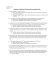### Past Final Exam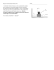### Physics 111 SI Worksheet 4 1. A 20 kg box sits on a horizontal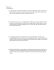### Free-Body Diagram Worksheet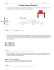### Vector Components Worksheet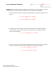### Homework 11 Problems – Simple Harmonic Motion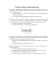### ENS1101 - Engineering Mechanics Assignment Lecturer: Dr Shuo LI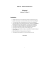### AC Geo/Adv.Alg Unit 1 Worksheet 11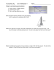### Inverse Trig Functions You know how to find the missing side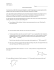### installation of an angle board * * * thermopian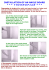### How to install RCS/RK TAGHeuer By Chronelec active loop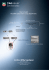### Worksheet D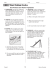### 9-8 Projectile Motion Virtual lab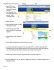### Test A - Physics 218